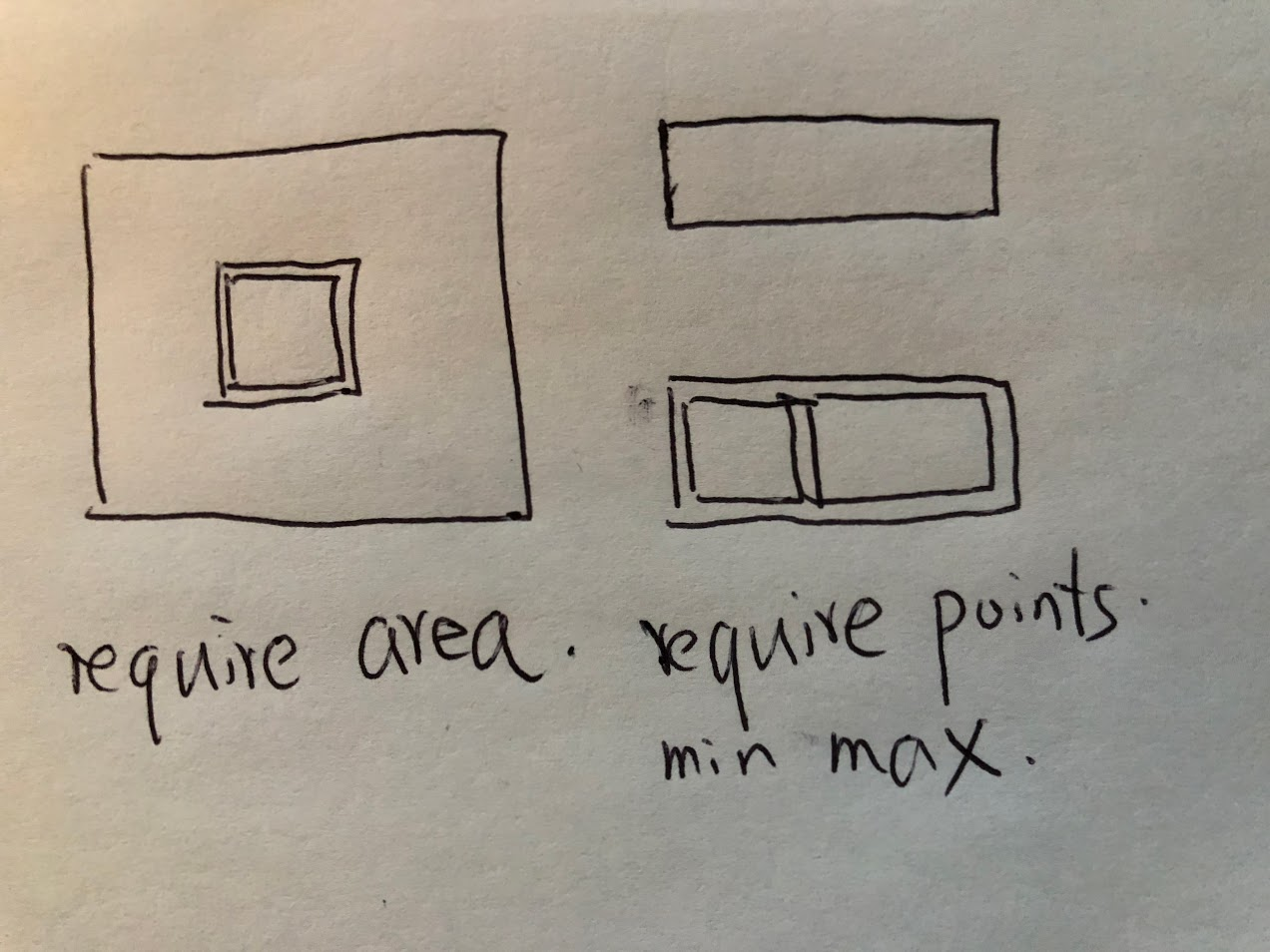# 391. Perfect Rectangle

### Problem:

Given N axis-aligned rectangles where N > 0, determine if they all together form an exact cover of a rectangular region.

Each rectangle is represented as a bottom-left point and a top-right point. For example, a unit square is represented as [1,1,2,2]. (coordinate of bottom-left point is (1, 1) and top-right point is (2, 2)).

Example 1:

``````rectangles = [
[1,1,3,3],
[3,1,4,2],
[3,2,4,4],
[1,3,2,4],
[2,3,3,4]
]

Return true. All 5 rectangles together form an exact cover of a rectangular region.
``````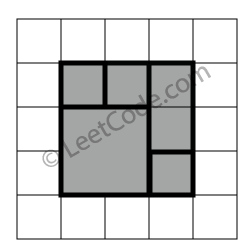Example 2:

``````rectangles = [
[1,1,2,3],
[1,3,2,4],
[3,1,4,2],
[3,2,4,4]
]

Return false. Because there is a gap between the two rectangular regions.
``````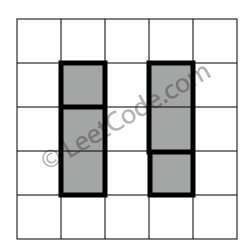Example 3:

``````rectangles = [
[1,1,3,3],
[3,1,4,2],
[1,3,2,4],
[3,2,4,4]
]

Return false. Because there is a gap in the top center.
``````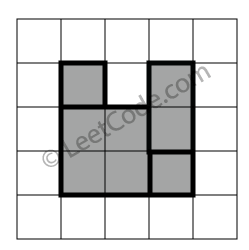Example 4:

``````rectangles = [
[1,1,3,3],
[3,1,4,2],
[1,3,2,4],
[2,2,4,4]
]

Return false. Because two of the rectangles overlap with each other.
``````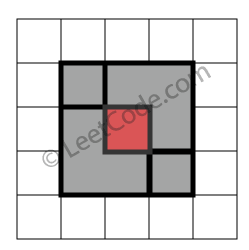### Solutions:

Not working solution

``````public class Solution {
public boolean isRectangleCover(int[][] rectangles) {
int blox = Integer.MAX_VALUE, bloy = Integer.MAX_VALUE, trix = Integer.MIN_VALUE, triy = Integer.MIN_VALUE;
for (int i = 0; i < rectangles.length; i ++) {
int[] rect = rectangles[i];
if (!(rect > blox || rect > bloy)) {
blox = rect;
bloy = rect;
}
if (!(rect < trix || rect < triy)) {
trix = rect;
triy = rect;
}
}

System.out.println(blox + ", " + bloy + " : " + trix + ", " + triy);
boolean[][] covered = new boolean[trix - blox][triy - bloy];
for (int i = 0; i < rectangles.length; i ++) {
int[] rect = rectangles[i];
for (int m = rect - blox; m < rect - blox; m ++) {
for (int n = rect - bloy; n < rect - bloy; n ++) {
if (m < 0 || m >= covered.length || n < 0 || n >= covered.length) {
System.out.println("out of bound");
return false;
}
if (covered[m][n] == true) {
return false;
}
covered[m][n] = true;
}
}
}
for (int i = 0; i < covered.length; i ++) {
for (int j = 0; j < covered.length; j ++) {
if (covered[i][j] == false) {
return false;
}
}
}
return true;
}
}
``````

Working solution

``````public class Solution {
public boolean isRectangleCover(int[][] rectangles) {
int blox = Integer.MAX_VALUE, bloy = Integer.MAX_VALUE, trix = Integer.MIN_VALUE, triy = Integer.MIN_VALUE;
int area = 0;
HashMap<String, Integer> data = new HashMap<String, Integer>();
for (int i = 0; i < rectangles.length; i ++) {
int[] rect = rectangles[i];
blox = Math.min(blox, rect);
bloy = Math.min(bloy, rect);
trix = Math.max(trix, rect);
triy = Math.max(triy, rect);
area += (rect - rect) * (rect - rect);
if (!isValid(data, rect + "," + rect, 1)) {
return false;
}
if (!isValid(data, rect + "," + rect, 2)) {
return false;
}
if (!isValid(data, rect + "," + rect, 4)) {
return false;
}
if (!isValid(data, rect + "," + rect, 8)) {
return false;
}
}
int count = 0;
for (String s:data.keySet()) {
int val = data.get(s);
if (val == 1 || val == 2 || val == 4 || val == 8) {
count ++;
}
}
return count == 4 && (trix - blox) * (triy - bloy) == area;
}
private boolean isValid(HashMap<String, Integer> data, String key, int mask) {
if (data.containsKey(key) && (data.get(key)&mask) != 0) {
return false;
}
int val = 0;
if (data.containsKey(key)) {
val = data.get(key);
}
data.put(key, val);
return true;
}
}
``````

Another working solutions.

``````public class Solution {
public boolean isRectangleCover(int[][] rectangles) {
int blox = Integer.MAX_VALUE, bloy = Integer.MAX_VALUE, trix = Integer.MIN_VALUE, triy = Integer.MIN_VALUE;
int area = 0;
Set<String> points = new HashSet<String>();
for (int i = 0; i < rectangles.length; i ++) {
int[] rect = rectangles[i];
blox = Math.min(blox, rect);
bloy = Math.min(bloy, rect);
trix = Math.max(trix, rect);
triy = Math.max(triy, rect);
area += (rect - rect) * (rect - rect);
String[] ps = new String;
ps = rect + "," + rect;
ps = rect + "," + rect;
ps = rect + "," + rect;
ps = rect + "," + rect;
for (int j = 0; j < ps.length; j ++) {
if (points.contains(ps[j])) {
points.remove(ps[j]);
}
else {
}
}
}
String[] ps = new String;
ps = blox + "," + bloy;
ps = blox + "," + triy;
ps = trix + "," + bloy;
ps = trix + "," + triy;
for (int i = 0; i < ps.length; i ++) {
if (!points.contains(ps[i])) {
return false;
}
}
return points.size() == 4 && (trix - blox) * (triy - bloy) == area;
}
}
``````